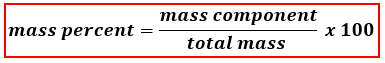# Problem: In a sample consisting of 1.00 mol NaBr and 0.300 mol KI, what is the mass percent of iodine?

###### FREE Expert Solution

We are asked to calculate for the percent by mass (mass %) of Iodinein this mixture and to do that we’re going to use the mass percent formula shown below:.

First, get the moles of I. We do that by doing a mole to mole comparison using KI. We see that for every mole of KI we have 1 mole of I.

99% (370 ratings)###### Problem Details

In a sample consisting of 1.00 mol NaBr and 0.300 mol KI, what is the mass percent of iodine?